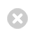Close

MPHY0021: Research Software Engineering With Python

Home## Recap example: Monte-Carlo¶

### Problem: Implement and test a simple Monte-Carlo algorithm¶

Given an input function (energy) and starting point (density) and a temperature $T$:

1. Compute energy at current density.
2. Move randomly chosen agent randomly left or right.
3. Compute second energy.
4. Compare the two energies:
5. If second energy is lower, accept move.
6. $\beta$ is a parameter which determines how likely the simulation is to move from a 'less favourable' situation to a 'more favourable' one.
7. Compute $P_0=e^{-\beta (E_1 - E_0)}$ and $P_1$ a uniformly distributed random number between 0 and 1,
8. If $P_0 > P_1$, do the move anyway.
9. Repeat.
• the algorithm should work for (m)any energy function(s).
• there should be separate tests for separate steps! What constitutes a step?
• tests for the Monte-Carlo should not depend on other parts of code.
• Use matplotlib to plot density at each iteration, and make an animation

### Solution¶

We need to break our problem down into pieces:

1. A function to generate a random change: random_agent(), random_direction()
2. A function to compute the energy before the change and after it: energy()
3. A function to determine the probability of a change given the energy difference (1 if decreases, otherwise based on exponential): change_density()
4. A function to determine whether to execute a change or not by drawing a random numberaccept_change()
5. A method to iterate the above procedure: step()

Next Step: Think about the possible unit tests

1. Input insanity: e.g. density should non-negative integer; testing by giving negative values etc.
2. change_density(): density is change by a particle hopping left or right? Do all positions have an equal chance of moving?
3. accept_change() will move be accepted when second energy is lower?
4. Make a small test case for the main algorithm. (Hint: by using mocking, we can pre-set who to move where.)
In :
%%bash
mkdir -p DiffusionExample

In :
%%writefile DiffusionExample/MonteCarlo.py
import matplotlib.pyplot as plt
from numpy import sum, array
from numpy.random import randint, choice

class MonteCarlo(object):
""" A simple Monte Carlo implementation """

def __init__(self, energy, density, temperature=1, itermax=1000):
from numpy import any, array
density = array(density)
self.itermax = itermax

if temperature == 0:
raise NotImplementedError(
"Zero temperature not implemented")
if temperature < 0e0:
raise ValueError(
"Negative temperature makes no sense")

if len(density) < 2:
raise ValueError("Density is too short")
# of the right kind (integer). Unless it is zero length,
# in which case type does not matter.
if density.dtype.kind != 'i' and len(density) > 0:
raise TypeError("Density should be an array of *integers*.")
# and the right values (positive or null)
if any(density < 0):
raise ValueError("Density should be an array of" +
"*positive* integers.")
if density.ndim != 1:
raise ValueError("Density should be an a *1-dimensional*" +
"array of positive integers.")
if sum(density) == 0:
raise ValueError("Density is empty.")

self.current_energy = energy(density)
self.temperature = temperature
self.density = density

def random_direction(self): return choice([-1, 1])

def random_agent(self, density):
# Particle index
particle = randint(sum(density))
current = 0
for location, n in enumerate(density):
current += n
if current > particle:
break
return location

def change_density(self, density):
""" Move one particle left or right. """

location = self.random_agent(density)

# Move direction
if(density[location]-1 < 0):
return array(density)
if location == 0:
direction = 1
elif location == len(density) - 1:
direction = -1
else:
direction = self.random_direction()

# Now make change
result = array(density)
result[location] -= 1
result[location + direction] += 1
return result

def accept_change(self, prior, successor):
""" Returns true if should accept change. """
from numpy import exp
from numpy.random import uniform
if successor <= prior:
return True
else:
return exp(-(successor - prior) / self.temperature) > uniform()

def step(self):
iteration = 0
while iteration < self.itermax:
new_density = self.change_density(self.density)
new_energy = energy(new_density)

accept = self.accept_change(self.current_energy, new_energy)
if accept:
self.density, self.current_energy = new_density, new_energy
iteration += 1

return self.current_energy, self.density

def energy(density, coefficient=1):
""" Energy associated with the diffusion model
:Parameters:
density: array of positive integers
Number of particles at each position i in the array/geometry
"""
from numpy import array, any, sum

# Make sure input is an array
density = array(density)

# of the right kind (integer). Unless it is zero length, in which case type does not matter.
if density.dtype.kind != 'i' and len(density) > 0:
raise TypeError("Density should be an array of *integers*.")
# and the right values (positive or null)
if any(density < 0):
raise ValueError("Density should be an array" +
"of *positive* integers.")
if density.ndim != 1:
raise ValueError("Density should be an a *1-dimensional*" +
"array of positive integers.")

return coefficient * 0.5 * sum(density * (density - 1))

Writing DiffusionExample/MonteCarlo.py

In :
import sys
sys.path.append('DiffusionExample')
from MonteCarlo import MonteCarlo, energy
import numpy as np
import numpy.random as random
from matplotlib import animation
from matplotlib import pyplot as plt
from IPython.display import HTML

Temperature = 0.1
density = [np.sin(i) for i in np.linspace(0.1, 3, 100)]
density = np.array(density)*100
density = density.astype(int)

fig = plt.figure()
ax = plt.axes(xlim=(-1, len(density)), ylim=(0, np.max(density)+1))
image = ax.scatter(range(len(density)), density)

txt_energy = plt.text(0, 100, 'Energy = 0')
plt.xlabel('Temperature = 0.1')
plt.ylabel('Energy Density')

mc = MonteCarlo(energy, density, temperature=Temperature)

def simulate(step):
energy, density = mc.step()
image.set_offsets(np.vstack((range(len(density)), density)).T)
txt_energy.set_text('Energy = {}'.format(energy))

anim = animation.FuncAnimation(fig, simulate, frames=200,
interval=50)
HTML(anim.to_jshtml())

Out: